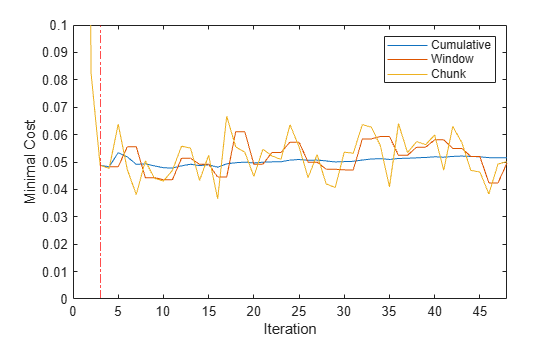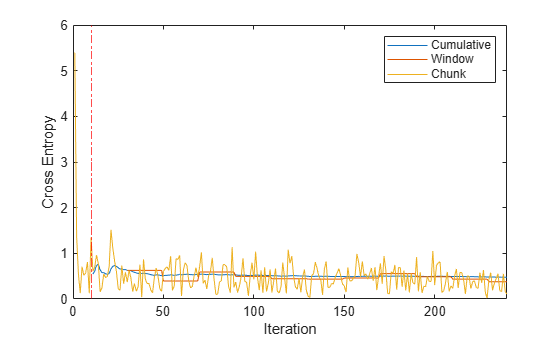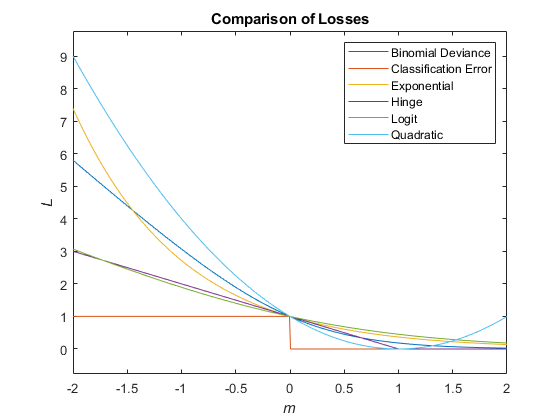# loss

Loss of naive Bayes incremental learning classification model on batch of data

## Syntax

``L = loss(Mdl,X,Y)``
``L = loss(Mdl,X,Y,Name,Value)``

## Description

`loss` returns the classification loss of a configured naive Bayes classification model for incremental learning (`incrementalClassificationNaiveBayes` object).

To measure model performance on a data stream and store the results in the output model, call `updateMetrics` or `updateMetricsAndFit`.

example

``L = loss(Mdl,X,Y)` returns the minimal cost classification loss for the naive Bayes classification model for incremental learning `Mdl` using the batch of predictor data `X` and corresponding responses `Y`.`

example

````L = loss(Mdl,X,Y,Name,Value)` uses additional options specified by one or more name-value arguments. For example, you can specify the classification loss function.```

## Examples

collapse all

Three different ways to measure performance of an incremental model on streaming data exist:

• Cumulative metrics measure the performance since the start of incremental learning.

• Window metrics measure the performance on a specified window of observations. The metrics are updated every time the model processes the specified window.

• The `loss` function measures the performance on a specified batch of data only.

Load the human activity data set. Randomly shuffle the data.

```load humanactivity n = numel(actid); rng(1) % For reproducibility idx = randsample(n,n); X = feat(idx,:); Y = actid(idx);```

For details on the data set, enter `Description` at the command line.

Create a naive Bayes classification model for incremental learning. Specify the class names and a metrics window size of 1000 observations. Configure the model for `loss` by fitting it to the first 10 observations.

```Mdl = incrementalClassificationNaiveBayes('ClassNames',unique(Y),'MetricsWindowSize',1000); initobs = 10; Mdl = fit(Mdl,X(1:initobs,:),Y(1:initobs)); canComputeLoss = (size(Mdl.DistributionParameters,2) == Mdl.NumPredictors) + ... (size(Mdl.DistributionParameters,1) > 1) > 1```
```canComputeLoss = logical 1 ```

`Mdl` is an `incrementalClassificationNaiveBayes` model. All its properties are read-only.

Simulate a data stream, and perform the following actions on each incoming chunk of 500 observations:

1. Call `updateMetrics` to measure the cumulative performance and the performance within a window of observations. Overwrite the previous incremental model with a new one to track performance metrics.

2. Call `loss` to measure the model performance on the incoming chunk.

3. Call `fit` to fit the model to the incoming chunk. Overwrite the previous incremental model with a new one fitted to the incoming observations.

4. Store all performance metrics to see how they evolve during incremental learning.

```% Preallocation numObsPerChunk = 500; nchunk = floor((n - initobs)/numObsPerChunk); mc = array2table(zeros(nchunk,3),'VariableNames',["Cumulative" "Window" "Chunk"]); % Incremental learning for j = 1:nchunk ibegin = min(n,numObsPerChunk*(j-1) + 1 + initobs); iend = min(n,numObsPerChunk*j + initobs); idx = ibegin:iend; Mdl = updateMetrics(Mdl,X(idx,:),Y(idx)); mc{j,["Cumulative" "Window"]} = Mdl.Metrics{"MinimalCost",:}; mc{j,"Chunk"} = loss(Mdl,X(idx,:),Y(idx)); Mdl = fit(Mdl,X(idx,:),Y(idx)); end```

Now, `Mdl` is an `incrementalClassificationNaiveBayes` model object trained on all the data in the stream. During incremental learning and after the model is warmed up, `updateMetrics` checks the performance of the model on the incoming observations, and then the `fit` function fits the model to those observations. `loss` is agnostic of the metrics warm-up period, so it measures the minimal cost for every chunk.

To see how the performance metrics evolve during training, plot them.

```figure plot(mc.Variables) xlim([0 nchunk]) ylim([0 0.1]) ylabel('Minimal Cost') xline(Mdl.MetricsWarmupPeriod/numObsPerChunk + 1,'r-.') legend(mc.Properties.VariableNames) xlabel('Iteration')```The yellow line represents the minimal cost on each incoming chunk of data. After the metrics warm-up period, `Mdl` tracks the cumulative and window metrics.

Fit a naive Bayes classification model for incremental learning to streaming data, and compute the multiclass cross entropy loss on the incoming chunks of data.

Load the human activity data set. Randomly shuffle the data.

```load humanactivity n = numel(actid); rng(1); % For reproducibility idx = randsample(n,n); X = feat(idx,:); Y = actid(idx);```

For details on the data set, enter `Description` at the command line.

Create a naive Bayes classification model for incremental learning. Configure the model as follows:

• Specify the class names.

• Specify a metrics warm-up period of 1000 observations.

• Specify a metrics window size of 2000 observations.

• Track the multiclass cross entropy loss to measure the performance of the model. Create an anonymous function that measures the multiclass cross entropy loss of each new observation, and include a tolerance for numerical stability. Create a structure array containing the name `CrossEntropy` and its corresponding function handle.

• Compute the classification loss by fitting the model to the first 10 observations.

```tolerance = 1e-10; crossentropy = @(z,zfit,w,cost)-log(max(zfit(z),tolerance)); ce = struct("CrossEntropy",crossentropy); Mdl = incrementalClassificationNaiveBayes('ClassNames',unique(Y),'MetricsWarmupPeriod',1000, ... 'MetricsWindowSize',2000,'Metrics',ce); initobs = 10; Mdl = fit(Mdl,X(1:initobs,:),Y(1:initobs));```

`Mdl` is an `incrementalClassificationNaiveBayes` model object configured for incremental learning.

Perform incremental learning. At each iteration:

• Simulate a data stream by processing a chunk of 100 observations.

• Call `updateMetrics` to compute cumulative and window metrics on the incoming chunk of data. Overwrite the previous incremental model with a new one fitted to overwrite the previous metrics.

• Call `loss` to compute the cross entropy on the incoming chunk of data. Whereas the cumulative and window metrics require that custom losses return the loss for each observation, `loss` requires the loss for the entire chunk. Compute the mean of the losses within a chunk.

• Call `fit` to fit the incremental model to the incoming chunk of data.

• Store the cumulative, window, and chunk metrics to see how they evolve during incremental learning.

```% Preallocation numObsPerChunk = 100; nchunk = floor((n - initobs)/numObsPerChunk); tanloss = array2table(zeros(nchunk,3),'VariableNames',["Cumulative" "Window" "Chunk"]); % Incremental fitting for j = 1:nchunk ibegin = min(n,numObsPerChunk*(j-1) + 1 + initobs); iend = min(n,numObsPerChunk*j + initobs); idx = ibegin:iend; Mdl = updateMetrics(Mdl,X(idx,:),Y(idx)); tanloss{j,1:2} = Mdl.Metrics{"CrossEntropy",:}; tanloss{j,3} = loss(Mdl,X(idx,:),Y(idx),'LossFun',@(z,zfit,w,cost)mean(crossentropy(z,zfit,w,cost))); Mdl = fit(Mdl,X(idx,:),Y(idx)); end```

`Mdl` is an `incrementalClassificationNaiveBayes` model object trained on all the data in the stream. During incremental learning and after the model is warmed up, `updateMetrics` checks the performance of the model on the incoming observations, and then the `fit` function fits the model to those observations.

Plot the performance metrics to see how they evolve during incremental learning.

```figure h = plot(tanloss.Variables); xlim([0 nchunk]) ylabel('Cross Entropy') xline(Mdl.MetricsWarmupPeriod/numObsPerChunk,'r-.') xlabel('Iteration') legend(h,tanloss.Properties.VariableNames)```The plot suggests the following:

• `updateMetrics` computes the performance metrics after the metrics warm-up period only.

• `updateMetrics` computes the cumulative metrics during each iteration.

• `updateMetrics` computes the window metrics after processing 2000 observations (20 iterations).

• Because `Mdl` is configured to predict observations from the beginning of incremental learning, `loss` can compute the cross entropy on each incoming chunk of data.

## Input Arguments

collapse all

Naive Bayes classification model for incremental learning, specified as an `incrementalClassificationNaiveBayes` model object. You can create `Mdl` directly or by converting a supported, traditionally trained machine learning model using the `incrementalLearner` function. For more details, see the corresponding reference page.

You must configure `Mdl` to compute its loss on a batch of observations.

• If `Mdl` is a converted, traditionally trained model, you can compute its loss without any modifications.

• Otherwise, you must fit the input model `Mdl` to data that contains all expected classes. That is, `Mdl.DistributionParameters` must be a cell matrix with `Mdl.NumPredictors` columns and at least one row, where each row corresponds to each class name in `Mdl.ClassNames`.

Batch of predictor data with which to compute the loss, specified as an n-by-`Mdl.NumPredictors` floating-point matrix.

The length of the observation labels `Y` and the number of observations in `X` must be equal; `Y(j)` is the label of observation j (row) in `X`.

Data Types: `single` | `double`

Batch of labels with which to compute the loss, specified as a categorical, character, or string array; logical or floating-point vector; or cell array of character vectors.

The length of the observation labels `Y` and the number of observations in `X` must be equal; `Y(j)` is the label of observation j (row) in `X`.

If `Y` contains a label that is not a member of `Mdl.ClassNames`, `loss` issues an error. The data type of `Y` and `Mdl.ClassNames` must be the same.

Data Types: `char` | `string` | `cell` | `categorical` | `logical` | `single` | `double`

### Name-Value Arguments

Specify optional pairs of arguments as `Name1=Value1,...,NameN=ValueN`, where `Name` is the argument name and `Value` is the corresponding value. Name-value arguments must appear after other arguments, but the order of the pairs does not matter.

Before R2021a, use commas to separate each name and value, and enclose `Name` in quotes.

Example: `'LossFun','classiferror','Weights',W` specifies returning the misclassification error rate, and the observation weights `W`.

Cost of misclassifying an observation, specified as a value in the table, where c is the number of classes in `Mdl.ClassNames`. The specified value overrides the value of `Mdl.Cost`.

ValueDescription
c-by-c numeric matrix

`Cost(i,j)` is the cost of classifying an observation into class `j` when its true class is `i`, for classes `Mdl.ClassNames(i)` and `Mdl.ClassNames(j)`. In other words, the rows correspond to the true class and the columns correspond to the predicted class. For example, `Cost = [0 2;1 0]` applies double the penalty for misclassifying `Mdl.ClassNames(1)` than for misclassifying `Mdl.ClassNames(2)`.

Structure array

A structure array having two fields:

• `ClassNames` containing the class names, the same value as `Mdl.ClassNames`

• `ClassificationCosts` containing the cost matrix, as previously described.

Example: `Cost=struct('ClassNames',Mdl.ClassNames,'ClassificationCosts',[0 2; 1 0])`

Data Types: `single` | `double` | `struct`

Loss function, specified as a built-in loss function name or function handle.

The following table lists the built-in loss function names. You can specify more than one by using a string vector.

NameDescription
`"binodeviance"`Binomial deviance
`"classiferror"`Misclassification error rate
`"exponential"`Exponential
`"hinge"`Hinge
`"logit"`Logistic
`"mincost"`

Minimal expected misclassification cost

`"quadratic"`Quadratic

For more details, see Classification Loss.

To specify a custom loss function, use function handle notation. The function must have this form:

`lossval = lossfcn(C,S,W,Cost)`

• The output argument `lossval` is an n-by-1 floating-point vector, where n is the number of observations in `X`. The value in `lossval(j)` is the classification loss of observation `j`.

• You specify the function name (`lossfcn`).

• `C` is an n-by-K logical matrix with rows indicating the class to which the corresponding observation belongs. `K` is the number of distinct classes (`numel(Mdl.ClassNames)`, and the column order corresponds to the class order in the `ClassNames` property. Create `C` by setting `C(p,q)` = `1`, if observation `p` is in class `q`, for each observation in the specified data. Set the other element in row `p` to `0`.

• `S` is an n-by-K numeric matrix of predicted classification scores. `S` is similar to the `Posterior` output of `predict`, where rows correspond to observations in the data and the column order corresponds to the class order in the `ClassNames` property. `S(p,q)` is the classification score of observation `p` being classified in class `q`.

• `W` is an n-by-1 numeric vector of observation weights.

• `Cost` is a K-by-K numeric matrix of misclassification costs.

Example: `'LossFun',"classiferror"`

Example: `'LossFun',@lossfcn`

Data Types: `char` | `string` | `function_handle`

Prior class probabilities, specified as a value in this numeric vector. `Prior` has the same length as the number of classes in `Mdl.ClassNames`, and the order of the elements corresponds to the class order in `Mdl.ClassNames`. `loss` normalizes the vector so that the sum of the result is 1.

The specified value overrides the value of `Mdl.Prior`.

Data Types: `single` | `double`

Score transformation function describing how incremental learning functions transform raw response values, specified as a character vector, string scalar, or function handle. The specified value overrides the value of `Mdl.ScoreTransform`.

This table describes the available built-in functions for score transformation.

ValueDescription
`"doublelogit"`1/(1 + e–2x)
`"invlogit"`log(x / (1 – x))
`"ismax"`Sets the score for the class with the largest score to 1, and sets the scores for all other classes to 0
`"logit"`1/(1 + ex)
`"none"` or `"identity"`x (no transformation)
`"sign"`–1 for x < 0
0 for x = 0
1 for x > 0
`"symmetric"`2x – 1
`"symmetricismax"`Sets the score for the class with the largest score to 1, and sets the scores for all other classes to –1
`"symmetriclogit"`2/(1 + ex) – 1

Data Types: `char` | `string`

Chunk of observation weights, specified as a floating-point vector of positive values. `loss` weighs the observations in `X` with the corresponding values in `Weights`. The size of `Weights` must equal n, the number of observations in `X`.

By default, `Weights` is `ones(n,1)`.

For more details, including normalization schemes, see Observation Weights.

Data Types: `double` | `single`

## Output Arguments

collapse all

Classification loss, returned as a numeric scalar. `L` is a measure of model quality. Its interpretation depends on the loss function and weighting scheme.

collapse all

### Classification Loss

Classification loss functions measure the predictive inaccuracy of classification models. When you compare the same type of loss among many models, a lower loss indicates a better predictive model.

Consider the following scenario.

• L is the weighted average classification loss.

• n is the sample size.

• For binary classification:

• yj is the observed class label. The software codes it as –1 or 1, indicating the negative or positive class (or the first or second class in the `ClassNames` property), respectively.

• f(Xj) is the positive-class classification score for observation (row) j of the predictor data X.

• mj = yjf(Xj) is the classification score for classifying observation j into the class corresponding to yj. Positive values of mj indicate correct classification and do not contribute much to the average loss. Negative values of mj indicate incorrect classification and contribute significantly to the average loss.

• For algorithms that support multiclass classification (that is, K ≥ 3):

• yj* is a vector of K – 1 zeros, with 1 in the position corresponding to the true, observed class yj. For example, if the true class of the second observation is the third class and K = 4, then y2* = [`0 0 1 0`]′. The order of the classes corresponds to the order in the `ClassNames` property of the input model.

• f(Xj) is the length K vector of class scores for observation j of the predictor data X. The order of the scores corresponds to the order of the classes in the `ClassNames` property of the input model.

• mj = yj*f(Xj). Therefore, mj is the scalar classification score that the model predicts for the true, observed class.

• The weight for observation j is wj. The software normalizes the observation weights so that they sum to the corresponding prior class probability stored in the `Prior` property. Therefore,

`$\sum _{j=1}^{n}{w}_{j}=1.$`

Given this scenario, the following table describes the supported loss functions that you can specify by using the `LossFun` name-value argument.

Loss FunctionValue of `LossFun`Equation
Binomial deviance`'binodeviance'`$L=\sum _{j=1}^{n}{w}_{j}\mathrm{log}\left\{1+\mathrm{exp}\left[-2{m}_{j}\right]\right\}.$
Observed misclassification cost`'classifcost'`

$L=\sum _{j=1}^{n}{w}_{j}{c}_{{y}_{j}{\stackrel{^}{y}}_{j}},$

where ${\stackrel{^}{y}}_{j}$ is the class label corresponding to the class with the maximal score, and ${c}_{{y}_{j}{\stackrel{^}{y}}_{j}}$ is the user-specified cost of classifying an observation into class ${\stackrel{^}{y}}_{j}$ when its true class is yj.

Misclassified rate in decimal`'classiferror'`

$L=\sum _{j=1}^{n}{w}_{j}I\left\{{\stackrel{^}{y}}_{j}\ne {y}_{j}\right\},$

where I{·} is the indicator function.

Cross-entropy loss`'crossentropy'`

`'crossentropy'` is appropriate only for neural network models.

The weighted cross-entropy loss is

`$L=-\sum _{j=1}^{n}\frac{{\stackrel{˜}{w}}_{j}\mathrm{log}\left({m}_{j}\right)}{Kn},$`

where the weights ${\stackrel{˜}{w}}_{j}$ are normalized to sum to n instead of 1.

Exponential loss`'exponential'`$L=\sum _{j=1}^{n}{w}_{j}\mathrm{exp}\left(-{m}_{j}\right).$
Hinge loss`'hinge'`$L=\sum _{j=1}^{n}{w}_{j}\mathrm{max}\left\{0,1-{m}_{j}\right\}.$
Logit loss`'logit'`$L=\sum _{j=1}^{n}{w}_{j}\mathrm{log}\left(1+\mathrm{exp}\left(-{m}_{j}\right)\right).$
Minimal expected misclassification cost`'mincost'`

`'mincost'` is appropriate only if classification scores are posterior probabilities.

The software computes the weighted minimal expected classification cost using this procedure for observations j = 1,...,n.

1. Estimate the expected misclassification cost of classifying the observation Xj into the class k:

`${\gamma }_{jk}={\left(f{\left({X}_{j}\right)}^{\prime }C\right)}_{k}.$`

f(Xj) is the column vector of class posterior probabilities for the observation Xj. C is the cost matrix stored in the `Cost` property of the model.

2. For observation j, predict the class label corresponding to the minimal expected misclassification cost:

`${\stackrel{^}{y}}_{j}=\underset{k=1,...,K}{\text{argmin}}{\gamma }_{jk}.$`

3. Using C, identify the cost incurred (cj) for making the prediction.

The weighted average of the minimal expected misclassification cost loss is

`$L=\sum _{j=1}^{n}{w}_{j}{c}_{j}.$`

Quadratic loss`'quadratic'`$L=\sum _{j=1}^{n}{w}_{j}{\left(1-{m}_{j}\right)}^{2}.$

If you use the default cost matrix (whose element value is 0 for correct classification and 1 for incorrect classification), then the loss values for `'classifcost'`, `'classiferror'`, and `'mincost'` are identical. For a model with a nondefault cost matrix, the `'classifcost'` loss is equivalent to the `'mincost'` loss most of the time. These losses can be different if prediction into the class with maximal posterior probability is different from prediction into the class with minimal expected cost. Note that `'mincost'` is appropriate only if classification scores are posterior probabilities.

This figure compares the loss functions (except `'classifcost'`, `'crossentropy'`, and `'mincost'`) over the score m for one observation. Some functions are normalized to pass through the point (0,1).## Algorithms

collapse all

### Observation Weights

For each conditional predictor distribution, `loss` computes the weighted average and standard deviation.

If the prior class probability distribution is known (in other words, the prior distribution is not empirical), `loss` normalizes observation weights to sum to the prior class probabilities in the respective classes. This action implies that the default observation weights are the respective prior class probabilities.

If the prior class probability distribution is empirical, the software normalizes the specified observation weights to sum to 1 each time you call `loss`.

## Version History

Introduced in R2021a